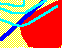7.1 OVERVIEW

In this chapter, we examine the interaction between demand and supply in the capital market. The interaction of the supply of and demand for financial assets (such as stocks) determines their prices. In practice, many assets are traded on exchanges, and prices arise from buyers and sellers agreeing to a price. The process of arriving at a price is sometimes called the price discovery process.

We describe a particular process called the competitive process. In describing this process, we start by asking: which portfolio would you choose, considering what you have learned about mean variance efficient portfolios? You will see that the capital market line (CML) considerably simplifies the answer to this question. Recall that the CML tells you the risk-return tradeoff in the capital market. Since efficient portfolios lie on the CML, they dominate any other portfolio. Thus, you, or any other investor satisfying the capital market assumptions we have made, would choose a portfolio on the CML.

But the CML is a straight line between the risk-free return and the point M. This means that any risk-return point on the CML can be obtained by holding only M and the risk-free asset. This result, called the two-fund theorem, means you would hold only some combination of M and the risk-free asset.

Therefore, it is important to find out exactly what M is. You will see that M can be given a quite simple interpretation. It is the value-weighted index of all risky securities, similar to the S&P 500 index, which we call the Market Portfolio.

This chapter has four main parts. The first part starts with Risk-Averse Investors, explains the individual choice problem, and describes preferences over risky assets. The second part is The CAPM in Equilibrium and it derives the two-fund theorem and explains why M is called the market portfolio. It ends with empirical tests of the CAPM. The third part contains a formal (and technical) derivation of the value of the firm in CAPM. Finally, the fourth part describes some corporate finance implications of the theory.

previous topic

next topic